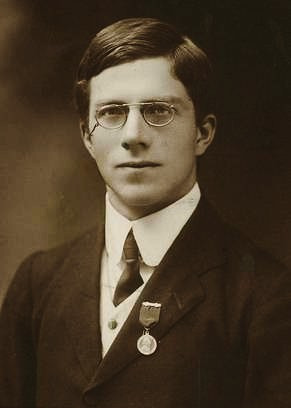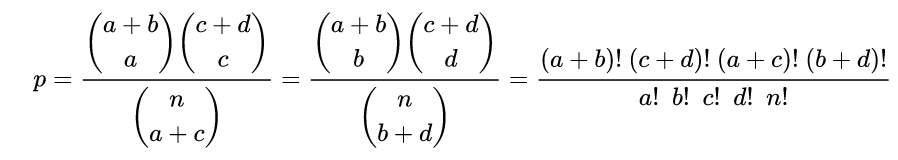Learn Biostatistics with Ria

Come on this incredible journey and help enhance your capability for Biomedical Research

# FISHER’S EXACT TEST

This is a variation of the Chi-Square test of association where we calculate the significance or p value from the exact probabilities of the categories. So no matter what the frequency is inside the contingency table (even 5 or less), we can calculate the exact p value and say whether the association between the two categorical variables are significant or not.

In case you are a little rusty about probability, here are some simple reminders:

(1) ! = factorial A factorial is the product of all of the whole numbers, except zero, that are less than or equal to that number.

(2) Whenever there are n number of things, and you are told to calculate the number of ways of choosing k number of items, then you can calculate like:
(nCk)= n! / k!(n-k)!

(3) Fisher's exact test is based on the hypergeometric distribution.

Let us understand this with an example: A medical clinic has 30 patients, 20 women and 10 men. A random sample of 5 patients is drawn. What is the probability that there will be 2 men?

A sample of 5 patients out of 30 can be chosen in (30C5) ways = 142,506 ways.

A sample of 2 men and 3 women can be drawn in (10C2)*(20C3) = 51,300 ways.

Therefore the Probability of choosing 2 men and 3 women are:

[ (10C2)*(20C3) ] / (30C5) = 51,300/142,506 = 0.359985.

Now that we have brushed up on the question of probability, let us move on to the Contingency table and see how Fisher’s Exact Test is related to this. Sir Ronald Aylmer Fisher, FRS (17 February 1890 – 29 July 1962) was a British polymath and biologist who was active as a mathematician, statistician, geneticist, and academic. He is said to single-handedly created the foundations for modern statistical science. This test is so called because the significance of the deviation from a Null hypothesis i.e. p-value can be calculated exactly, rather than relying on an approximation that becomes exact in the limit as the sample size grows to infinity, as with many statistical tests.

# Sir Ronald Aylmer Fisher, FRSIn Fisher's original example, one criterion of classification was whether milk or tea was put in the cup first; the other could be whether Bristol (someone he knows) thought that the milk or tea was put in first. We want to know whether these two classifications are associated—i.e. whether Bristol really can tell whether milk or tea was poured in first. The p-value from the test is computed as if the margins of the table are fixed. Bristol knows the number of cups with each treatment (milk or tea first) and will therefore provide guesses with the correct number in each category. As pointed out by Fisher, this leads under a Null hypothesis of independence to a hypergeometric distribution of the numbers in the cells of the table.

# Fisher's original example of tea and milkFisher’s exact test can be used on the following conditions:

• when expected frequencies are < 1 in a 2 x 2 chi square table

• when the number of observations is ≤ 20 in a 2 x 2 chi square table

Although in practice it is employed when sample sizes are small, it is valid for all sample sizes.

 Habit of smoking TOTAL Gender Smoker Non-smoker Male a b a + b Female c d c + d TOTAL a + c b + d n

In a scenario usually, we are given with (a + b) number of males and (c + d) number of females, in n number of people. Now I want to know, if a random sample of (a + c) is drawn, how many of them would be males. Following the previous example, we can write like this:The Fisher’s exact p is: p = [(a + b)! (c + d)! (a + c)! (b + d)!] / [n!a!b!c!d!]

Conclusion: During reporting of the results, the usual Chi-square value should be reported along with degree of freedom, followed by the Fisher’s Exact p value, instead of the approximated p-value. We would conclude that the distribution in the observed 2 x 2 table at the .05 level is statistically significant and different from chance. Thus, we can reject Null Hypothesis and say that there is a statistically significant association between the two categorical variables.

BIBLIOGRAPHY:

1. Anders Hald. A history of mathematical statistics from 1750 to 1930. the University of Michigan (1988)

2. Julien I.E. Hoffman. Hypergeometric Distribution. Biostatistics for Medical and Biomedical Practitioners. Academic Press. (2015). p:179-182. Link: doi.org/10.1016/B978-0-12-802387-7.00013-5.

# NEXT UP

Independent t-test

Written by:

Dr. Ria Roy
Senior Resident
Department of Community and Family Medicine, AIIMS Patna

Interests: Adolescent Health, Nutrition, Biostatistics, Epidemiology, NCDsLaunch your Graphy
100K+ creators trust Graphy to teach online